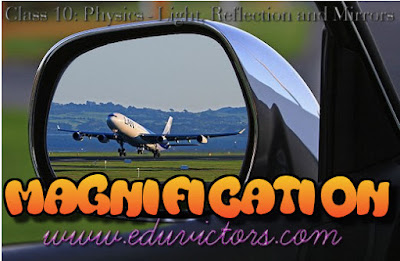## Light and Reflection - Magnification Chart For Mirrors

Q: What is Magnification?

Answer: The ratio of height of image to the height of an object is known as magnification of an image and is denoted as m.

```           Image height         h'     -v
m = -------------------- = ---- = ----
Object height        h       u

```

The +ve value of magnification represents virtual image of an object.

The -ve value of magnification represents real image of an object.

Q: Using magnification formula, prove that image formed by a plane mirror is at the same distance as object but behind the mirror. It is given that in plane mirror, object height and image height are equal.

Answer: In plane mirror h' = h

```     h'        -v
m = --- = 1 =  ---
h          u

⇒ v = -u

```

∴ image formed by a plane mirror is at the same distance as object but behind the mirror.

Magnification and Type of Image and Mirror

Magnification(m) Type of image and its size Type of Mirror
+1 Virtual erect and of same size as an object Plane
-1 Real inverted and of same size as an object. Concave
>1 and -ve Real inverted and magnified Concave
< 1 and -ve Real inverted and diminished Concave
> 1 and positive Virtual, erect and magnified Concave
< and positive Virtual, erect and diminished Convex

Q: The magnification of a mirror is -2. Height of the object is 15cm. What is the height of the image? What type of image will it be?

Answer: Given m = -2, h = 15cm, h' = ?
h' = m ✕ h = -2 ✕ 15cm = -30cm

It forms real inverted and enlarged image.